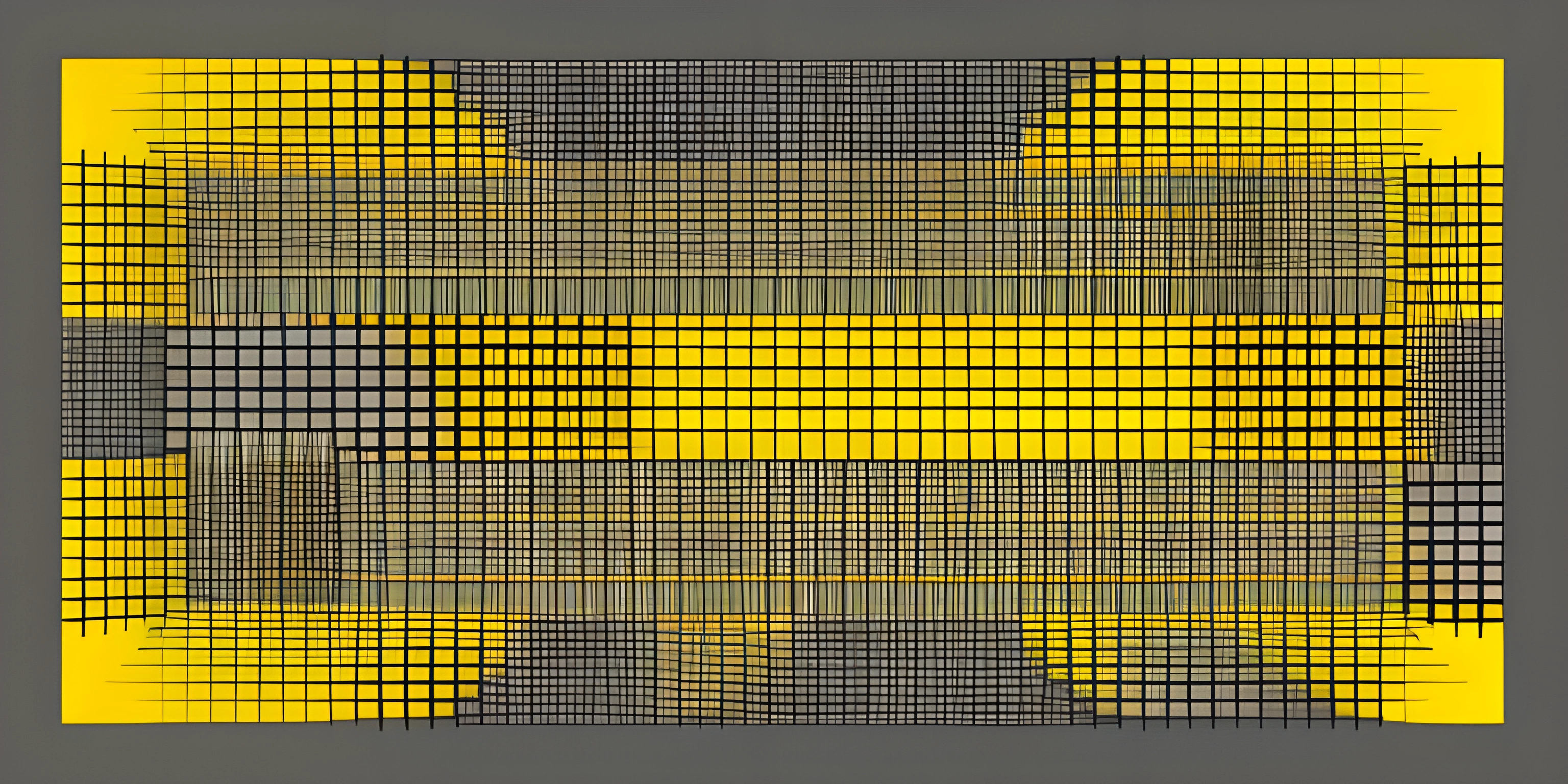# Working with Matrices in MATLABNote: this page has been created with the use of AI. Please take caution, and note that the content of this page does not necessarily reflect the opinion of Cratecode.

When working with MATLAB, it's often essential to work with matrices. These two-dimensional arrays are at the heart of the language, and MATLAB provides a robust and versatile selection of tools for creating, manipulating, and performing operations with them. So buckle up and let's dive into the world of matrices in MATLAB!

## Creating Matrices

To create a matrix in MATLAB, you can simply list its elements within square brackets and separate them with spaces for columns and semicolons for rows. For example, creating a 3x3 matrix would look like this:

``A = [1 2 3; 4 5 6; 7 8 9]``

This will create a matrix A:

``````1  2  3
4  5  6
7  8  9``````

### Special Matrices

MATLAB also provides functions to generate specific types of matrices. Some commonly used functions include:

• `zeros(m, n)`: Creates an m-by-n matrix filled with zeros.
• `ones(m, n)`: Creates an m-by-n matrix filled with ones.
• `eye(m, n)`: Creates an m-by-n identity matrix (ones on the diagonal and zeros elsewhere).

For example:

``````B = zeros(2, 3)
C = ones(3, 3)
D = eye(3)``````

## Accessing and Modifying Matrix Elements

To access an element of a matrix, you can use the row and column indices in parentheses:

``element = A(2, 3)  % Access the element in the 2nd row and 3rd column``

To modify a matrix element, simply assign a new value to the desired index:

``A(1, 1) = 42;``

### Accessing Rows and Columns

You can also access entire rows or columns by using the colon `:` operator:

``````row = A(2, :)
column = A(:, 3)``````

## Matrix Operations

MATLAB makes it easy to perform operations on matrices. Here are some common operations:

To add or subtract matrices, use the `+` and `-` operators, respectively:

``````E = A + C
F = A - C``````

### Multiplication

To multiply matrices, use the `*` operator:

``G = A * D``

Keep in mind that this is true matrix multiplication, not element-wise multiplication. For element-wise multiplication, use the `.*` operator:

``H = A .* C``

### Division

Matrix division is more complex than scalar division. In MATLAB, you can perform left division using the `\` operator and right division using the `/` operator:

``````I = A / C
J = A \ C``````

### Transpose

To transpose a matrix, use the `'` operator:

``K = A'``

## FAQ

### How do I create a matrix in MATLAB?

You can create a matrix in MATLAB by listing its elements within square brackets and separating them with spaces for columns and semicolons for rows, like this: `A = [1 2 3; 4 5 6; 7 8 9]`.

### What are some common matrix operations in MATLAB?

Some common matrix operations include addition, subtraction, multiplication, division, and transposing. MATLAB provides operators and functions for all these operations, making it simple to work with matrices.

### How do I access individual elements, rows, or columns of a matrix in MATLAB?

To access an individual element, use row and column indices in parentheses, like this: `element = A(2, 3)`. To access a row or column, use the colon `:` operator, like this: `row = A(2, :)` or `column = A(:, 3)`.

### How do I perform element-wise multiplication in MATLAB?

To perform element-wise multiplication, use the `.*` operator, like this: `H = A .* C`.